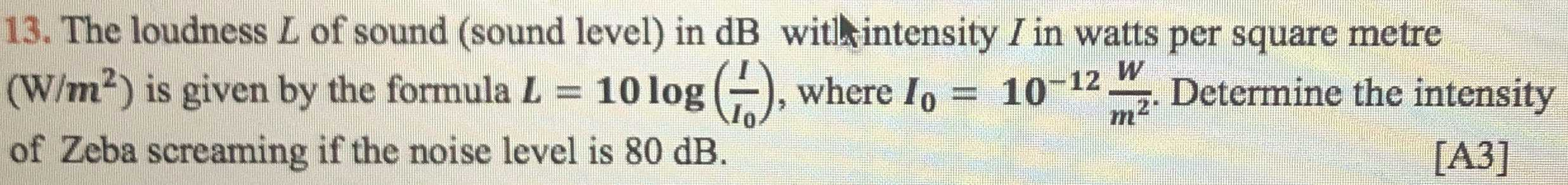### ¿Todavía tienes preguntas de matemáticas?

Pregunte a nuestros tutores expertos
Algebra
Pregunta13. The loudness $$L$$ of sound (sound level) in $$dB$$ witl hintensity $$I$$ in watts per square metre

$$( W / m ^ { 2 }$$ ) is given by the formula $$L = 10 \log ( \frac { I } { I _ { 0 } } )$$ , where $$I _ { 0 } = 10 ^ { - 12 } \frac { W } { m ^ { 2 } }$$ . Determine the intensity of Zeba screaming if the noise level is $$80 dB$$ .

I = 10$$^{- 4}$$ (W/m$$^2$$)